Publicité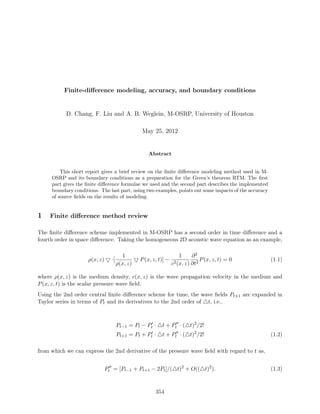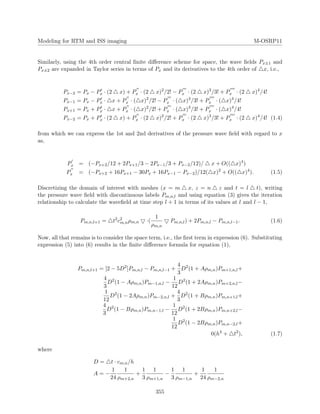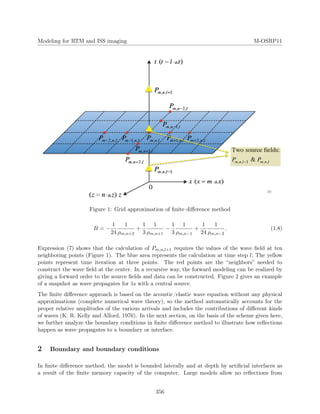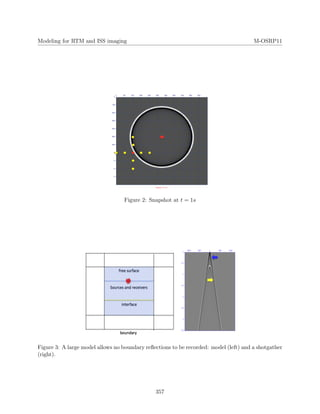Publicité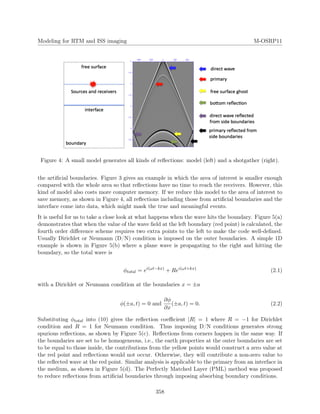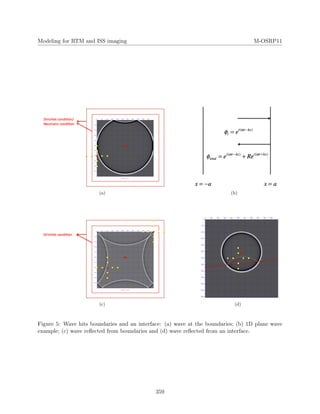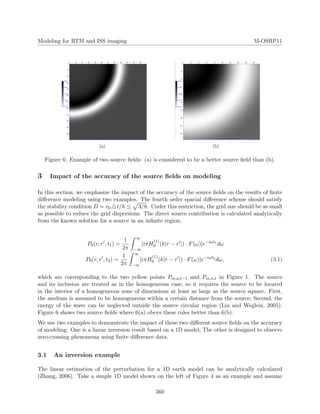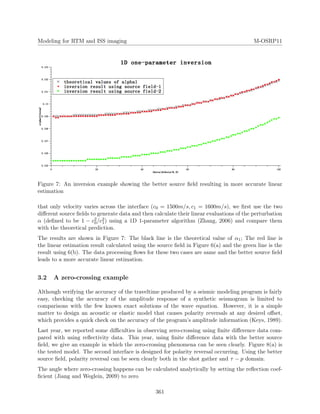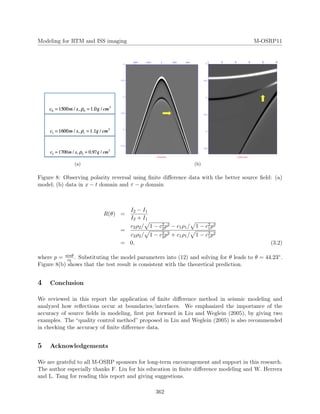Publicité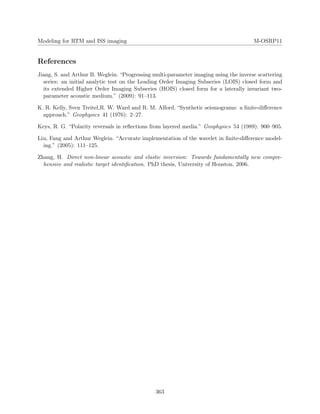Prochain SlideShare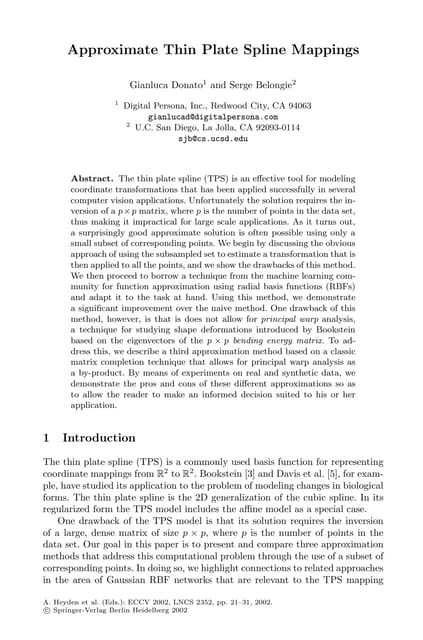Approximate Thin Plate Spline Mappings
Chargement dans ... 3
1 sur 10
Publicité

### Chang etal 2012a

1. Finite-diﬀerence modeling, accuracy, and boundary conditions D. Chang, F. Liu and A. B. Weglein, M-OSRP, University of Houston May 25, 2012 Abstract This short report gives a brief review on the ﬁnite diﬀerence modeling method used in M- OSRP and its boundary conditions as a preparation for the Green’s theorem RTM. The ﬁrst part gives the ﬁnite diﬀerence formulae we used and the second part describes the implemented boundary conditions. The last part, using two examples, points out some impacts of the accuracy of source ﬁelds on the results of modeling. 1 Finite diﬀerence method review The ﬁnite diﬀerence scheme implemented in M-OSRP has a second order in time diﬀerence and a fourth order in space diﬀerence. Taking the homogeneous 2D acoustic wave equation as an example, ρ(x, z) ·[ 1 ρ(x, z) P(x, z, t)] − 1 c2(x, z) ∂2 ∂t2 P(x, z, t) = 0 (1.1) where ρ(x, z) is the medium density, c(x, z) is the wave propagation velocity in the medium and P(x, z, t) is the scalar pressure wave ﬁeld. Using the 2nd order central ﬁnite diﬀerence scheme for time, the wave ﬁelds Pt±1 are expanded in Taylor series in terms of Pt and its derivatives to the 2nd order of t, i.e., Pt−1 = Pt − Pt · t + Pt · ( t)2 /2! Pt+1 = Pt + Pt · t + Pt · ( t)2 /2! (1.2) from which we can express the 2nd derivative of the pressure wave ﬁeld with regard to t as, Pt = [Pt−1 + Pt+1 − 2Pt]/( t)2 + O(( t)2 ). (1.3) 354
2. Modeling for RTM and ISS imaging M-OSRP11 Similarly, using the 4th order central ﬁnite diﬀerence scheme for space, the wave ﬁelds Px±1 and Px±2 are expanded in Taylor series in terms of Px and its derivatives to the 4th order of x, i.e., Px−2 = Px − Px · (2 x) + Px · (2 x)2 /2! − Px · (2 x)3 /3! + Px · (2 x)4 /4! Px−1 = Px − Px · x + Px · ( x)2 /2! − Px · ( x)3 /3! + Px · ( x)4 /4! Px+1 = Px + Px · x + Px · ( x)2 /2! + Px · ( x)3 /3! + Px · ( x)4 /4! Px−2 = Px + Px · (2 x) + Px · (2 x)2 /2! + Px · (2 x)3 /3! + Px · (2 x)4 /4! (1.4) from which we can express the 1st and 2nd derivatives of the pressure wave ﬁeld with regard to x as, Px = (−Px+2/12 + 2Px+1/3 − 2Px−1/3 + Px−2/12)/ x + O(( x)4 ) Px = (−Px+2 + 16Px+1 − 30Px + 16Px−1 − Px−2)/12( x)2 + O(( x)4 ). (1.5) Discretizing the domain of interest with meshes (x = m x, z = n z and t = l t), writing the pressure wave ﬁeld with discontinuous labels Pm,n,l and using equation (3) gives the iteration relationship to calculate the waveﬁeld at time step l + 1 in terms of its values at l and l − 1, Pm,n,l+1 = t2 c2 m,nρm,n ·( 1 ρm,n Pm,n,l) + 2Pm,n,l − Pm,n,l−1. (1.6) Now, all that remains is to consider the space term, i.e., the ﬁrst term in expression (6). Substituting expression (5) into (6) results in the ﬁnite diﬀerence formula for equation (1), Pm,n,l+1 = [2 − 5D2 ]Pm,n,l − Pm,n,l−1 + 4 3 D2 (1 + Aρm,n)Pm+1,n,l+ 4 3 D2 (1 − Aρm,n)Pm−1,n,l − 1 12 D2 (1 + 2Aρm,n)Pm+2,n,l− 1 12 D2 (1 − 2Aρm,n)Pm−2,n,l + 4 3 D2 (1 + Bρm,n)Pm,n+1,l+ 4 3 D2 (1 − Bρm,n)Pm,n−1,l − 1 12 D2 (1 + 2Bρm,n)Pm,n+2,l− 1 12 D2 (1 − 2Bρm,n)Pm,n−2,l+ 0(h4 + t2 ), (1.7) where D = t · cm,n/h A = − 1 24 1 ρm+2,n + 1 3 1 ρm+1,n − 1 3 1 ρm−1,n + 1 24 1 ρm−2,n 355
3. Modeling for RTM and ISS imaging M-OSRP11 Figure 1: Grid approximation of ﬁnite diﬀerence method B = − 1 24 1 ρm,n+2 + 1 3 1 ρm,n+1 − 1 3 1 ρm,n−1 + 1 24 1 ρm,n−2 . (1.8) Expression (7) shows that the calculation of Pm,n,l+1 requires the values of the wave ﬁeld at ten neighboring points (Figure 1). The blue area represents the calculation at time step l; The yellow points represent time iteration at three points. The red points are the “neighbors” needed to construct the wave ﬁeld at the center. In a recursive way, the forward modeling can be realized by giving a forward order to the source ﬁelds and data can be constructed. Figure 2 gives an example of a snapshot as wave propagates for 1s with a central source. The ﬁnite diﬀerence approach is based on the acoustic/elastic wave equation without any physical approximations (complete numerical wave theory), so the method automatically accounts for the proper relative amplitudes of the various arrivals and includes the contributions of diﬀerent kinds of waves (K. R. Kelly and Alford, 1976). In the next section, on the basis of the scheme given here, we further analyze the boundary conditions in ﬁnite diﬀerence method to illustrate how reﬂections happen as wave propagates to a boundary or interface. 2 Boundary and boundary conditions In ﬁnite diﬀerence method, the model is bounded laterally and at depth by artiﬁcial interfaces as a result of the ﬁnite memory capacity of the computer. Large models allow no reﬂections from 356
4. Modeling for RTM and ISS imaging M-OSRP11 Figure 2: Snapshot at t = 1s Figure 3: A large model allows no boundary reﬂections to be recorded: model (left) and a shotgather (right). 357
5. Modeling for RTM and ISS imaging M-OSRP11 Figure 4: A small model generates all kinds of reﬂections: model (left) and a shotgather (right). the artiﬁcial boundaries. Figure 3 gives an example in which the area of interest is smaller enough compared with the whole area so that reﬂections have no time to reach the receivers. However, this kind of model also costs more computer memory. If we reduce this model to the area of interest to save memory, as shown in Figure 4, all reﬂections including those from artiﬁcial boundaries and the interface come into data, which might mask the true and meaningful events. It is useful for us to take a close look at what happens when the wave hits the boundary. Figure 5(a) demonstrates that when the value of the wave ﬁeld at the left boundary (red point) is calculated, the fourth order diﬀerence scheme requires two extra points to the left to make the code well-deﬁned. Usually Dirichlet or Neumann (D/N) condition is imposed on the outer boundaries. A simple 1D example is shown in Figure 5(b) where a plane wave is propagating to the right and hitting the boundary, so the total wave is φtotal = ei(ωt−kx) + Rei(ωt+kx) (2.1) with a Dirichlet or Neumann condition at the boundaries x = ±a φ(±a, t) = 0 and ∂φ ∂x (±a, t) = 0. (2.2) Substituting φtotal into (10) gives the reﬂection coeﬃcient |R| = 1 where R = −1 for Dirichlet condition and R = 1 for Neumann condition. Thus imposing D/N conditions generates strong spurious reﬂections, as shown by Figure 5(c). Reﬂections from corners happen in the same way. If the boundaries are set to be homogeneous, i.e., the earth properties at the outer boundaries are set to be equal to those inside, the contributions from the yellow points would construct a zero value at the red point and reﬂections would not occur. Otherwise, they will contribute a non-zero value to the reﬂected wave at the red point. Similar analysis is applicable to the primary from an interface in the medium, as shown in Figure 5(d). The Perfectly Matched Layer (PML) method was proposed to reduce reﬂections from artiﬁcial boundaries through imposing absorbing boundary conditions. 358
6. Modeling for RTM and ISS imaging M-OSRP11 (a) (b) (c) (d) Figure 5: Wave hits boundaries and an interface: (a) wave at the boundaries; (b) 1D plane wave example; (c) wave reﬂected from boundaries and (d) wave reﬂected from an interface. 359
7. Modeling for RTM and ISS imaging M-OSRP11 (a) (b) Figure 6: Example of two source ﬁelds: (a) is considered to be a better source ﬁeld than (b). 3 Impact of the accuracy of the source ﬁelds on modeling In this section, we emphasize the impact of the accuracy of the source ﬁelds on the results of ﬁnite diﬀerence modeling using two examples. The fourth order spacial diﬀerence scheme should satisfy the stability condition D = c0 t/h ≤ 3/8. Under this restriction, the grid size should be as small as possible to reduce the grid dispersions. The direct source contribution is calculated analytically from the known solution for a source in an inﬁnite region, P0(r, r , t1) = 1 2π ∞ −∞ [iπH (1) 0 (k|r − r |) · F(ω)]e−iωt1 dω P0(r, r , t2) = 1 2π ∞ −∞ [iπH (1) 0 (k|r − r |) · F(ω)]e−iωt2 dω, (3.1) which are corresponding to the two yellow points Pm,n,l−1 and Pm,n,l in Figure 1. The source and its inclusion are treated as in the homogeneous case, so it requires the source to be located in the interior of a homogeneous zone of dimensions at least as large as the source square. First, the medium is assumed to be homogeneous within a certain distance from the source; Second, the energy of the wave can be neglected outside the source circular region (Liu and Weglein, 2005). Figure 6 shows two source ﬁelds where 6(a) obeys these rules better than 6(b). We use two examples to demonstrate the impact of these two diﬀerent source ﬁelds on the accuracy of modeling: One is a linear inversion result based on a 1D model; The other is designed to observe zero-crossing phenomena using ﬁnite diﬀerence data. 3.1 An inversion example The linear estimation of the perturbation for a 1D earth model can be analytically calculated (Zhang, 2006). Take a simple 1D model shown on the left of Figure 4 as an example and assume 360
8. Modeling for RTM and ISS imaging M-OSRP11 Figure 7: An inversion example showing the better source ﬁeld resulting in more accurate linear estimation that only velocity varies across the interface (c0 = 1500m/s, c1 = 1600m/s), we ﬁrst use the two diﬀerent source ﬁelds to generate data and then calculate their linear evaluations of the perturbation α (deﬁned to be 1 − c2 0/c2 1) using a 1D 1-parameter algorithm (Zhang, 2006) and compare them with the theoretical prediction. The results are shown in Figure 7: The black line is the theoretical value of α1; The red line is the linear estimation result calculated using the source ﬁeld in Figure 6(a) and the green line is the result using 6(b). The data processing ﬂows for these two cases are same and the better source ﬁeld leads to a more accurate linear estimation. 3.2 A zero-crossing example Although verifying the accuracy of the traveltime produced by a seismic modeling program is fairly easy, checking the accuracy of the amplitude response of a synthetic seismogram is limited to comparisons with the few known exact solutions of the wave equation. However, it is a simple matter to design an acoustic or elastic model that causes polarity reversals at any desired oﬀset, which provides a quick check on the accuracy of the program’s amplitude information (Keys, 1989). Last year, we reported some diﬃculties in observing zero-crossing using ﬁnite diﬀerence data com- pared with using reﬂectivity data. This year, using ﬁnite diﬀerence data with the better source ﬁeld, we give an example in which the zero-crossing phenomena can be seen clearly. Figure 8(a) is the tested model. The second interface is designed for polarity reversal occurring. Using the better source ﬁeld, polarity reversal can be seen clearly both in the shot gather and τ − p domain. The angle where zero-crossing happens can be calculated analytically by setting the reﬂection coef- ﬁcient (Jiang and Weglein, 2009) to zero 361
9. Modeling for RTM and ISS imaging M-OSRP11 (a) (b) Figure 8: Observing polarity reversal using ﬁnite diﬀerence data with the better source ﬁeld: (a) model; (b) data in x − t domain and τ − p domain R(θ) = I2 − I1 I2 + I1 = c2ρ2/ 1 − c2 2p2 − c1ρ1/ 1 − c2 1p2 c2ρ2/ 1 − c2 2p2 + c1ρ1/ 1 − c2 1p2 = 0, (3.2) where p = sinθ c0 . Substituting the model parameters into (12) and solving for θ leads to θ = 44.23◦. Figure 8(b) shows that the test result is consistent with the theoretical prediction. 4 Conclusion We reviewed in this report the application of ﬁnite diﬀerence method in seismic modeling and analyzed how reﬂections occur at boundaries/interfaces. We emphasized the importance of the accuracy of source ﬁelds in modeling, ﬁrst put forward in Liu and Weglein (2005), by giving two examples. The “quality control method” proposed in Liu and Weglein (2005) is also recommended in checking the accuracy of ﬁnite diﬀerence data. 5 Acknowledgements We are grateful to all M-OSRP sponsors for long-term encouragement and support in this research. The author especially thanks F. Liu for his education in ﬁnite diﬀerence modeling and W. Herrera and L. Tang for reading this report and giving suggestions. 362
10. Modeling for RTM and ISS imaging M-OSRP11 References Jiang, S. and Arthur B. Weglein. “Progressing multi-parameter imaging using the inverse scattering series: an initial analytic test on the Leading Order Imaging Subseries (LOIS) closed form and its extended Higher Order Imaging Subseries (HOIS) closed form for a laterally invariant two- parameter acoustic medium.” (2009): 91–113. K. R. Kelly, Sven Treitel,R. W. Ward and R. M. Alford. “Synthetic seismograms: a ﬁnite-diﬀerence approach.” Geophysics 41 (1976): 2–27. Keys, R. G. “Polarity reversals in reﬂections from layered media.” Geophysics 54 (1989): 900–905. Liu, Fang and Arthur Weglein. “Accurate implementation of the wavelet in ﬁnite-diﬀerence model- ing.” (2005): 111–125. Zhang, H. Direct non-linear acoustic and elastic inversion: Towards fundamentally new compre- hensive and realistic target identiﬁcation. PhD thesis, University of Houston, 2006. 363
Publicité